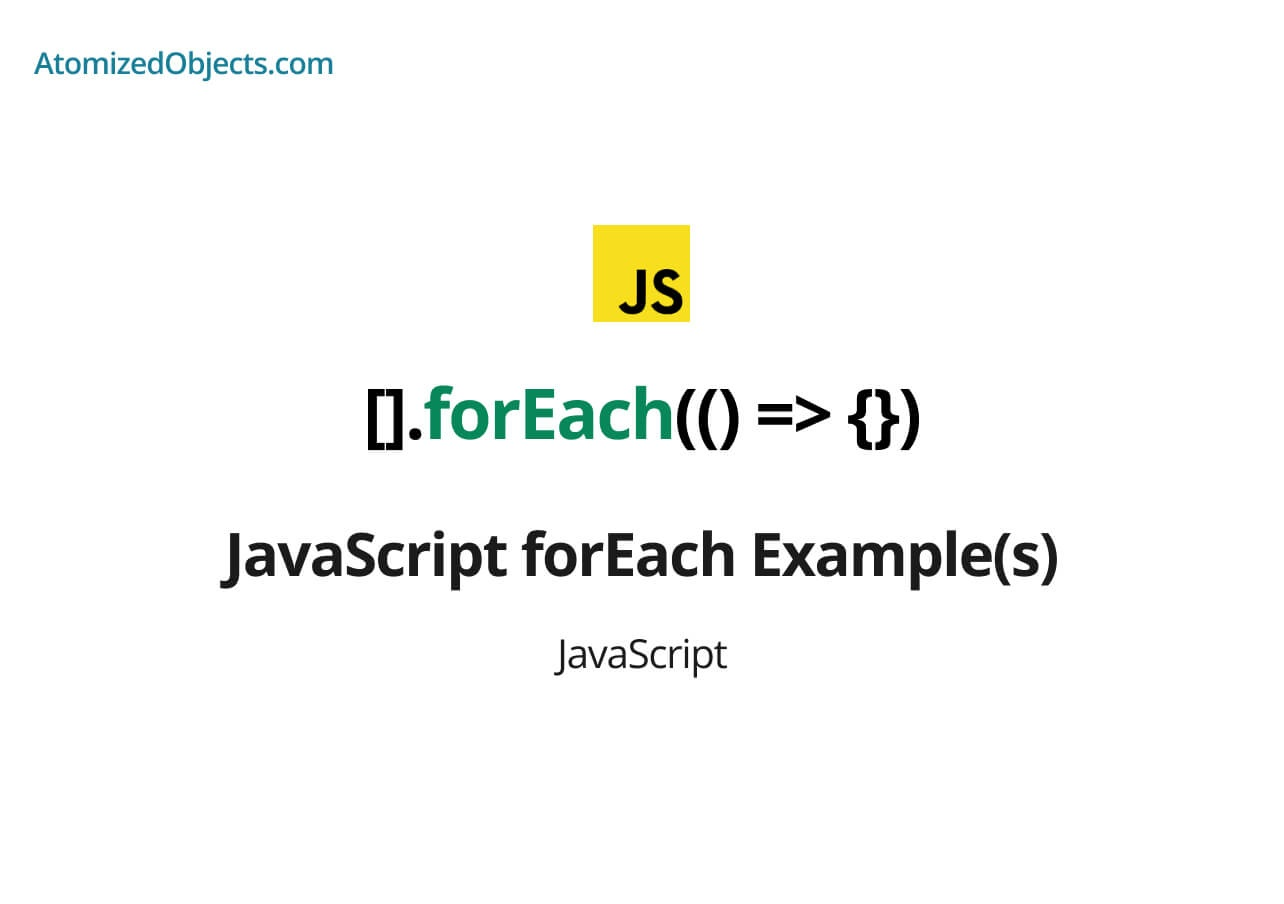Blog / Javascript

# JavaScript forEach Example(s)In this post we will look at all the javascript foreach example(s) that you need in order to reference and use the Array.prototype.forEach method in just about any case.Will Mayger
October 20, 2021
Article

In this post we will look at examples for most use cases of the JavaScript forEach method that you can use on arrays.

The Array.prototype.forEach method has many different uses but for the most part it is a simple and easy way to loop over an array instead of having to use a for loop, there are times however when a for loop is actually better suited but in this post we will look at each use case and examples as each so that you can use it to reference how to use the JavaScript forEach method.

## JavaScript forEach Example

Let’s start with the simplest example for the JavaScript forEach method which is to just call it on an array like so `[].forEach((value, index, fullArrray) => console.log(value, index, fullArray))`.

The forEach method is a way to loop and iterate over an array in JavaScript, to use it we just need to call forEach on an Array and provide a callback function that accepts a value, an index and a full array.

``````["Ted", "Bob", "Amy"].forEach((value, index, fullArray) => {
console.log(value); // "ted", "bob", "amy"
console.log(index); // 0, 1, 2
console.log(fullArray); // ["Ted", "Bob", "Amy"], ["Ted", "Bob", "Amy"], ["Ted", "Bob", "Amy"]
});``````

As you can see with the forEach method it accepts a callback function that is passed the value, the index and the full array for each iteration / loop of the array.

Building on this simple example, let’s take a look at a full use case / JavaScript forEach example:

``````function findAmountOfNumbersMatchingIndex(numbers) {
let numbersMatchingIndex = 0;

numbers.forEach((n, index) => {
if (n === index) {
numbersMatchingIndex += 1;
}
});

return numbersMatchingIndex;
}

findAmountOfNumbersMatchingIndex([1, 1, 3, 4]); // 1``````

### JavaScript forEach key value example & JavaScript forEach object example

Next up is the example of using the JavaScript Array.prototype.forEach method in combination with Object.entries to get key value pairs in order to loop over an object.

To read more detail about using forEach with key value pairs I have a post that goes into detail about key value pairs with Object.entries and Array.prototype.forEach.

Here is the JavaScript forEach key value example and JavaScript forEach object example:

``````const animals = { cats: 2, dogs: 4 };

Object.entries(animals).forEach(([key, value], index, fullArray) => {
console.log(key, value); // "cats, 2", "dogs, 4"
console.log(index); // 0, 1
console.log(fullArray); // [["cats", 2], ["dogs", 4]]
});``````

As you can see the Objects.entries method converts an object into an array containing key value pairs in the form of nested arrays.

We can then combine this with the JavaScript forEach method to be able to loop over each whilst getting the key value pairs.

Another option is to use Object.keys and then use the key to reference the object for the value.

Here is an example of using Object.keys with forEach to get the key value pairs:

``````const animals = { cats: 2, dogs: 4 };

Object.keys(animals).forEach((key, index, fullArray) => {
const value = animals[key];
console.log(key, value); // "cats, 2", "dogs, 4"
console.log(index); // 0, 1
console.log(fullArray); // [["cats", 2], ["dogs", 4]]
});``````

### JavaScript forEach continue example

Even though technically you can’t use a continue statement in a forEach loop in JavaScript you can “continue” and skip to the next iteration by instead using the return statement.

For more detail on how to continue in a JavaScript forEach loop click here.

Now let’s checkout the JavaScript forEach continue example:

``````[1, 2, 3, 4].forEach(item => {
if (item < 2) {
return; // emulating JavaScript forEach continue statement
}
console.log(item);
});

// 3, 4``````

### Other resources

There are a few commonly asked questions about JavaScript forEach such as “JavaScript forEach async examples”, and “JavaScript forEach break examples”.

Unfortunately you can’t actually use forEach with async code, just like you can’t break a forEach loop like you can a for loop.

With that said, there are workarounds for both which I cover in detail in the following two posts:

### Summary

There we have most examples you will need to be able to use the JavaScript forEach method in most situations.

There we have javaScript forEach Example(s), if you want more like this be sure to check out some of my other posts!Foxi

Budget Planner & Tracker

More money in your pocket by the end of the month.

Free to use and no account needed.

Get started now.

Some graphics used on this post were made using icons from flaticon.